The NLIN Procedure

Confidence Intervals

Subsections:

Parameter Confidence Intervals

By default, the parameter confidence intervals are computed using the Wald-based formula: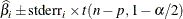where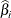is the ith parameter estimate,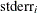is its estimated standard error,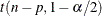is a t statistic with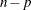degrees of freedom, n is the number of usable observations, and p is the number of parameters. You can specify the significance level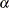used in constructing these confidence limits with the ALPHA= option in the PROC NLIN statement; the default value is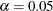.

Because Wald-based confidence intervals are only asymptotically valid, you can use the BOOTSTRAP statement to request bootstrap-based confidence intervals. Furthermore, you can augment these confidence intervals with confidence curves for a more complete inference on confidence intervals. For more information, see the section Bootstrap Resampling and Estimation and Profiling Parameters and Assessing the Influence of Observations on Parameter Estimates.

Model Confidence Intervals

Model confidence intervals are output when an OUT= data set is specified and one or more of the keywords LCLM , UCLM , LCL , UCL , L95M= , U95M= , L95= , and U95= is specified. The expressions for these terms are as follows: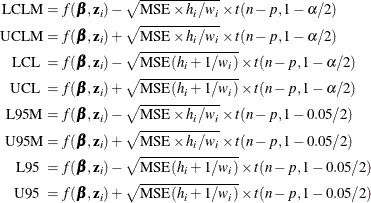where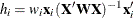is the leverage,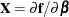, and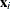is the ith row of. These results are derived for linear systems. The intervals are approximate for nonlinear models. The valuein the preceding formulas for LCLM , UCLM , LCL , and UCL can be set with the ALPHA= option in the PROC NLIN statement or with the ALPHA= option in the OUTPUT statement. If both ALPHA= options are specified, the option in the OUTPUT takes precedence.